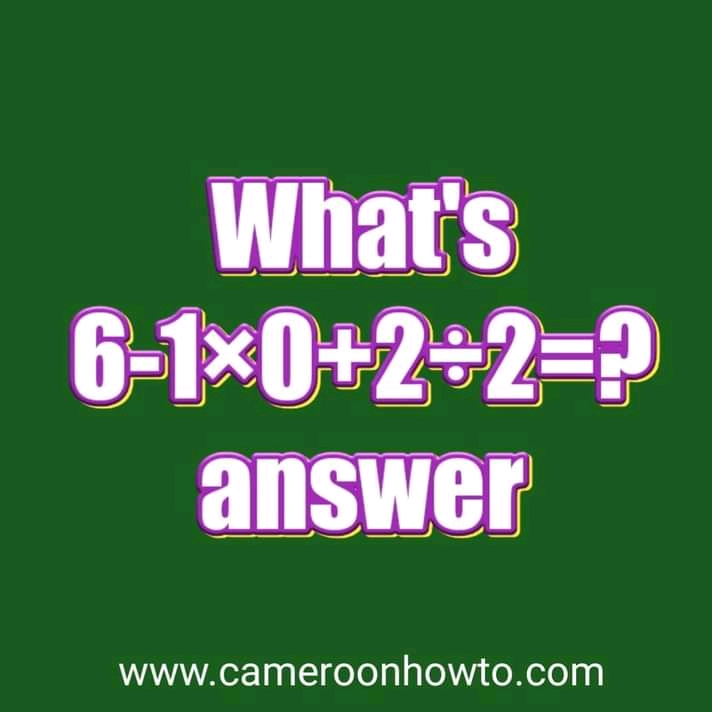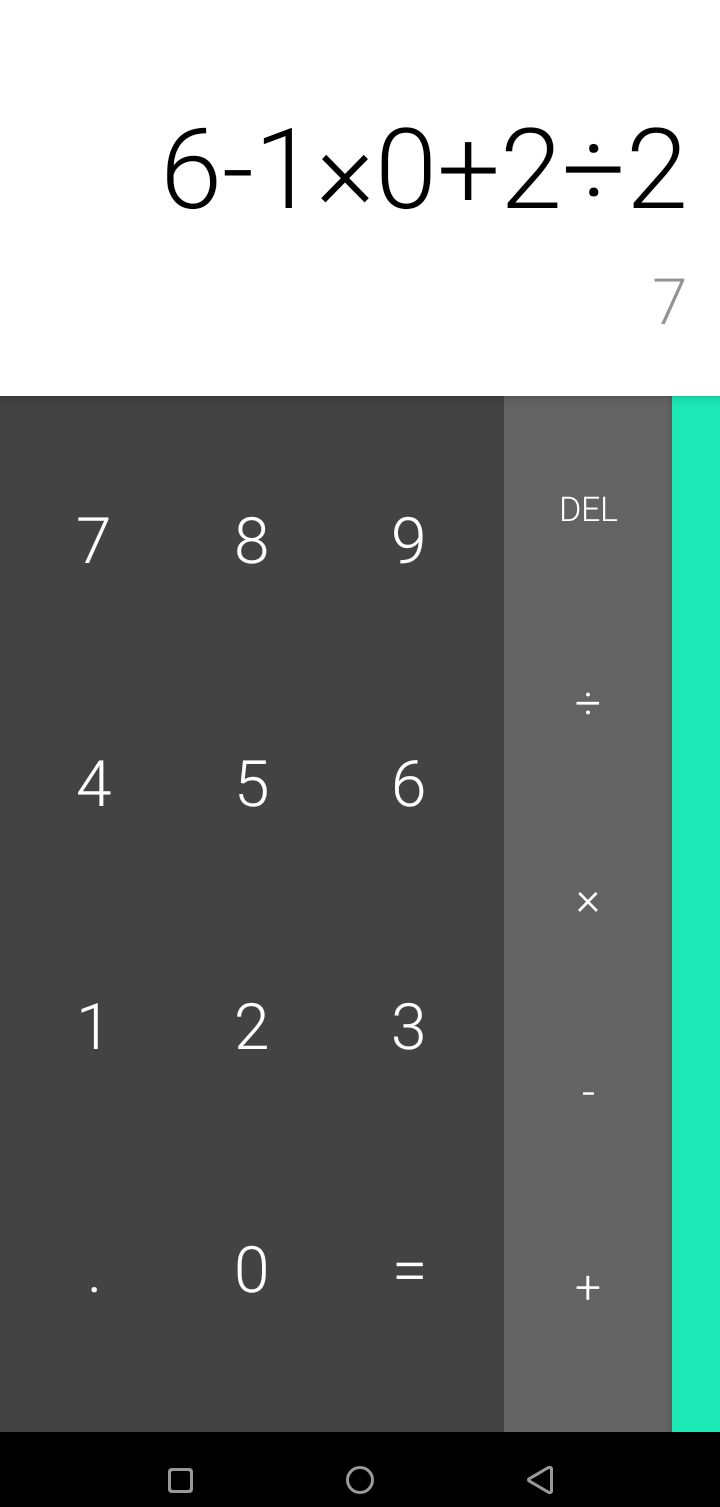# 6-1×0+2÷2= ? tricky math quiz answer ( details solution)

0

6-1×0+2÷2= ? tricky math quiz answer ( details solution)This tricky Math question has gone viral over the internet with many people having their answers as 0 and 7. Some have theirs which are not even near correct.

The correct answer to 6-1×0+2÷2= ? Would be 7 not 1, I will show you why

in solving below with the correct answer.

Also read: Tricky Math test 18 ÷ 3 (5 - 4 + 1) = ?H

Many game lovers like dare games, puzzles, sudoku quizzes etc. Solving tricky Math problems can refresh your brain and relax your mind.

6-1×0+2÷2= ? tricky math quiz answer ( details solution)

Solution

To solve this, all you need to do is to apply the concept of BODMAS OR PEMDAS which I have explained in other blog posts with good examples.

We have been using BODMAS since our primary school days but many of us have not understood how to apply it well to solve Math problems.

For example, the "O" in BODMAS stands for Order of operation and not "Of" as some people mistake it. If you still have doubts and want to know more details about BODMAS, then click here.

BODMAS is acronym which stands for

B: Bracket

O: Order of Operations

D: Division

M: Multiplication

S: Subtraction.

So, With BODMAS, B stands for bracket meaning we should solve whatever is in the bracket but since there's no Bracket, I follow the order of operations and the next is Division.

So, 2÷2= 1;

Rewriting the question will be as thus:

6-1×0+1=? . The "O" in BODMAS means Order of Operation and not "of" as some people mistake it. That's after you solve what is in the bracket, you need to follow a particular order too as you solve ( Divisional, Multiplication, Addition and Subtraction).

Solving continues

The next going by BODMAS is Multiplication.

So, -1×0= 0

So,6+0+1=?

7=?

Therefore, the correct answer is 6-1×0+2÷2= ? Math test is 7.

if you follow the BODMAS or PEMDAS role correctly.

See screenshot below on my calculator.

If your answer is 0, it means you considered the phrase: "Anything multiplied by zero is 0" and you didn't apply it well which is wrong.

To confirm the answer, I input the Quiz in a calculator and the answer is 7, check the screenshot below.Hopefully you learn something new today, if you have different views, then leave your comments below.